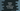# Python program to print all even numbers in a range## Python 3 program to print all even numbers in a range:

In this example, we will learn how to print all the even numbers in a given range. The even-number is a number that is perfectly divisible by 2 or the remainder is 0 _if you divide that number by _2. For finding out all even numbers in a given range, we need the start and the _end _point in the number series.

We will ask the user to enter these values. After that, we will iterate all the numbers in this range one by one. If an even number is found, our program will print it to the user.

Simple, isn’t it?

Steps we are going to use in this program are as below :

### Steps to find even numbers in a range :

1. Take the lower limit and upper limit from the user. Store the values in two separate variables.
2. Run one loop from lower limit to upper limit.
3. Check for each number if it is divisible by 2 or not on each iteration of the loop.
4. If it is divisible by 2, print out the number. Else, move to the next iteration.
5. Exit when the loop is completed.

### Python 3 Program :

``````lower_limit = int(input("Enter the lower limit : "))
upper_limit = int(input("Enter the upper limit : "))

for i in range(lower_limit,upper_limit+1):
if(i%2 == 0):
print(i)``````

### Explanation :

1. User input is read using the ‘input()’ method. As this is a number, it is converted to an integer by using the ‘int(input())’ method. ‘input()’ method returns the user entered data as a string. Using int(), we are converting it to an integer. Note that the user entered value should be an integer, or else it will throw one exception.
2. As explained above, we need to store the lower and upper range or limit in different variables to iterate in between them. In this example program, the lower limit is stored in_ ‘lowerlimit’ variable and the upper limit is saved in_ ‘upper_limit’ _variable.
3. We used a ‘for’ loop to iterate between the numbers here. You can also use one while loop.
4. ‘for i in range(lowerlimit,upperlimit+1)’ means the loop will run from ‘lowerlimit’_ to ‘upperlimit’. e.g. if _‘lowerlimit’_ is 1 and ‘upperlimit’_ is 3, the loop will run for i=1, i=2 and i=3. range() method takes two parameters. The first one is the lower limit for the loop and the second one is the upper limit for the loop. The upper limit is excluded and the lower limit is included in the loop iteration.
5. Inside the loop, we are checking if the number is divisible by 2 or not. For that, we are using ‘i%2′. ‘%’ or ‘modulo‘ operator returns the reminder . If the number is perfectly divisible by 2, it will return ‘0’. Modulo operator is the easiest way to test if a number is perfectly divisible by another number or not.
6. If the current number is perfectly divisible by 2, print out the number. Else move to the next iteration.

### Conclusion :

In this example, we have learned how to print all even numbers in a range in python. You can use the same approach to find out any types of numbers in a given range. Try to run the above example and drop one comment below if you have any queries.